# Axe’s matching algorithm¶

Axe uses an algorithm based on longest-prefix-in-trie matching to match a variable length from the start of each read against a set of ‘mutated’ indexes.

## Hamming distance matching¶

While for most applications in high-throughput sequencing hamming distances are a frowned-upon metric, it is typical for HTS read indexes to be designed to tolerate a certain level of hamming mismatches. Given these sequences are short and typically occur at the 5’ end of reads, insertions and deletions rarely need be considered, and the increased rate of assignment of reads with many errors is offset by the risk of falsely assigning indexes to an incorrect sample. In any case, reads with more than 1-2 sequencing errors in their first several bases are likely to be poor quality, and will simply be filtered out during downstream quality control.

## Hamming mismatch tries¶

Typically, reads are matched to a set of indexes by calculating the hamming distance between the index, and the firstbases of a read for a index of length. The “correct” index is then selected by recording either the index with the lowest hamming distance to the read (competitive matching) or by simply accepting the first index with a hamming distance below a certain threshold. These approaches are both very computationally expensive, and can have lower accuracy than the algorithm I propose. Additionally, implementations of these methods rarely handle indexes of differing length and combinatorial indexing well, if at all.

Central to Axe’s algorithm is the concept of hamming-mismatch tries. A trie is a N-ary tree for an N letter alphabet. In the case of high-throughput sequencing reads, we have the alphabet `AGCT`, corresponding to the four nucleotides of DNA, plus `N`, used to represent ambiguous base calls. Instead of matching each index to each read, we pre-calculate all allowable sequences at each mismatch level, and store these in level-wise tries. For example, to match to a hamming distance of 2, we create three tries: One containing all indexes, verbatim, and two tries where every sequence within a hamming distance of 1 and 2 of each index respectively. Hereafter, these tries are referred to as the 0, 1 and 2-mm tries, for a hamming distance (mismatch) of 0, 1 and 2. Then, we find the longest prefix in each sequence read in the 0mm trie. If this prefix is not a valid leaf in the 0mm trie, we find the longest prefix in the 1mm trie, and so on for all tries in ascending order. If no prefix of the read is a complete sequence in any trie, the read is assigned to an “non-indexed” output file.

This algorithm ensures optimal index matching in many ways, but is also extremely fast. In situations with indexes of differing length, we ensure that the longest acceptable index at a given hamming distance is chosen; assuming that sequence is random after the index, the probability of false assignments using this method is low. We also ensure that short perfect matches are preferred to longer inexact matches, as we firstly only consider indexes with no error, then 1 error, and so on. This ensures that reads with indexes that are followed by random sequence that happens to inexactly match a longer index in the set are not falsely assigned to this longer index.

The speed of this algorithm is largely due to the constant time matching algorithm with respect to the number of indexes to match. The time taken to match each read is proportional instead to the length of the indexes, as for a index of length, at most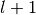trie level descents are required to find an entry in the trie. As this length is more-or-less constant and small, the overall complexity of axe’s algorithm is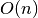for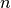reads, as opposed to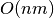forreads and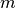indexes as is typical for traditional matching algorithms